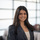# Regression Options to Analyze your Data

Oftentimes, we want to explore the relationship and correlation between multiple variables. Below are three different methods you can achieve this in Periscope, along with important tips to know as you're building out your models

Method 1: Scatter Plot Trendlines

We'll start with the easiest way to display a linear relationship - plotting a Scatter plot with a trendline, as shown below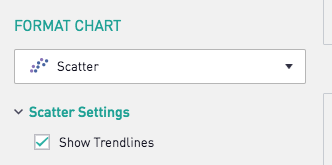Generating the following output: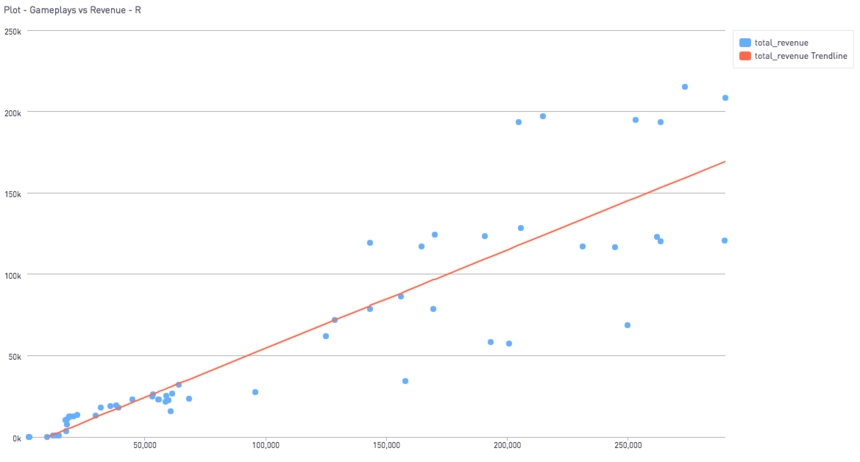The trendline is a Least Squares Regression Line. In other words, the line drawn minimizes the sum of the square of the residuals. While we were able to create this line with a simple click, we would need to harness the heavier computational power of the R and Python integration in order to view the coefficients of the trendline, assess the estimate error, and perform calculations using the trendline to generate useful data points (such a residuals). This leads us to methods 2 and 3.

Method 2: Linear Regression in Python

The community post here details how to perform Linear Regressions in Python. Note that the trendline here is based on a random 70% of the dataset. Depending on which 70% is selected, the trendline can vary slightly. In contrast, the trendline checkbox for Periscope scatter plots uses 100% of the data points to create a least squares regression line (thus it always displays the same line). This does not necessarily mean one model is better than the other. In fact, by using only 70% of the dataset to train a model in Python, we leave 30% of the dataset to test the model, giving us a glimpse of how effectively the model can be used to make predictions.

Python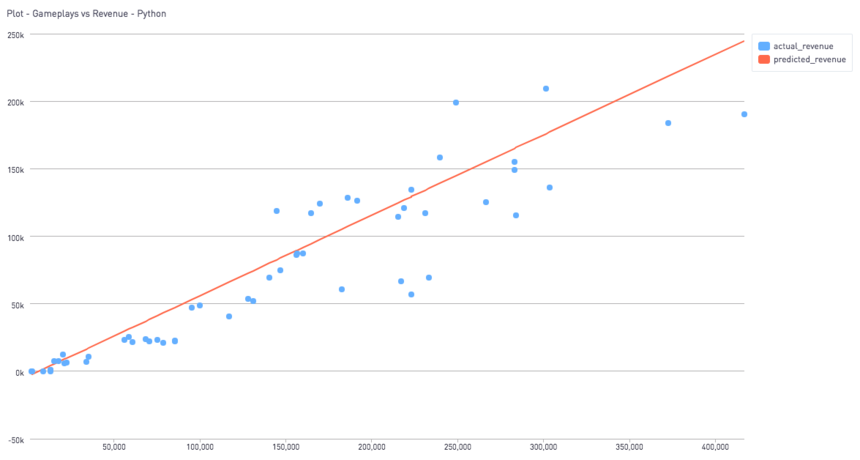Method 3: Linear Regression in R

Similar to Python, we can create a linear regression model in R. The methodology here also generates a linear model based on a random 70% of the full dataset, leaving the remaining 30% to test the data.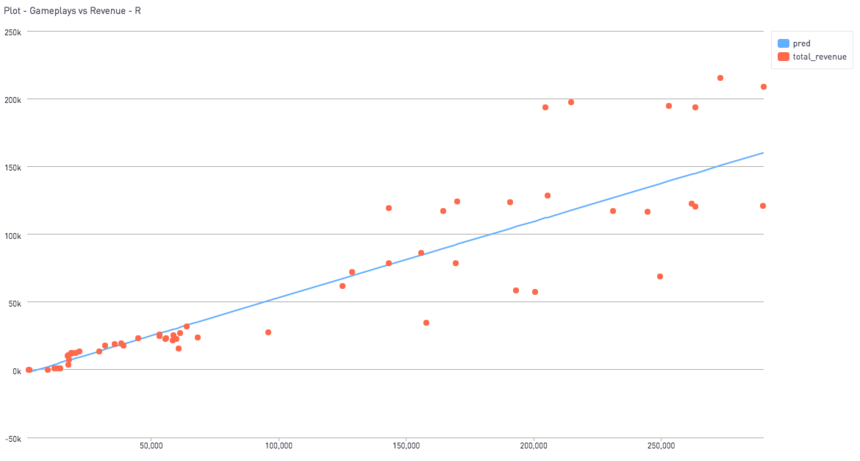Which Linear Regression model do you prefer? Comment below!